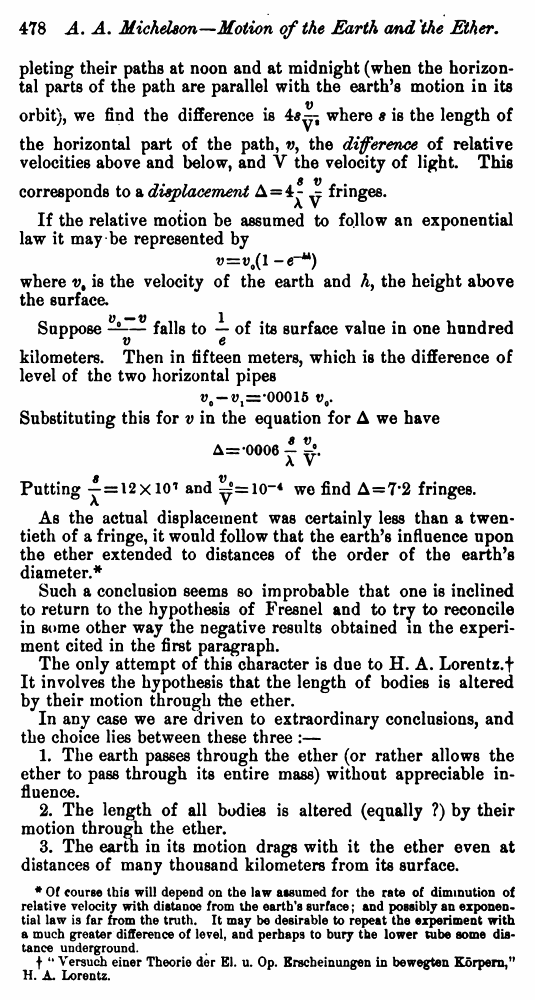Michelson A.A. The relative Motion of the Earth and the Ether. // American Journal of Science.—Fourth Series, Vol. III, No. 18.—June, 1897.

В начало   Другие форматы   <<<     Страница 478   >>>1  475  476  477  478 pleting their paths at noon and at midnight (when the horizontal parts of the path are parallel with the earth’s motion in its orbit), we find the difference iswhere s is the length of the horizontal part of the path, v, the difference of relative velocities above and below, and V the velocity of light. This corresponds to a displacementfringes. If the relative motion be assumed to follow an exponential law it may be represented by where v, is the velocity of the earth and h, the height above the surface. Supposefalls toof its surface value in one hundred kilometers. Then in fifteen meters, which is the difference of level of the two horizontal pipes Substituting this for v in the equation for Δ we have Puttingandwe find Δ=7.2 fringes. As the actual displacement was certainly less than a twentieth of a fringe, it would follow that the earth’s influence upon the ether extended to distances of the order of the earth’s diameter.* Such a conclusion seems so improbable that one is inclined to return to the hypothesis of Fresnel and to try to reconcile in some other way the negative results obtained in the experiment cited in the first paragraph. The only attempt of this character is due to H. A. Lorentz.† It involves the hypothesis that the length of bodies is altered by their motion through the ether. In any case we are driven to extraordinary conclusions, and the choice lies between these three :— 1. The earth passes through the ether (or rather allows the ether to pass through its entire mass) without appreciable influence. 2. The length of all bodies is altered (equally ?) by their motion through the ether. 3. The earth in its motion drags with it the ether even at distances of many thousand kilometers from its surface. * Of course this will depend on the law assumed for the rate of diminution of relative velocity with distanoe from the earth's surface; and possibly an exponential law is far from the truth. It may be desirable to repeat the experiment with a much greater difference of level, and perhaps to bury the lower tube some distance underground. † "Versuch einer Theorie der El. u. Op. Erecheinungen in bewegten Körpern,” H. A. Lorentz.